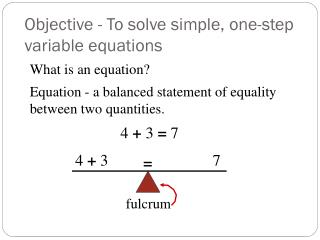# Objective - To solve simple, one-step variable equations - PowerPoint PPT PresentationDownload PresentationObjective - To solve simple, one-step variable equations

Objective - To solve simple, one-step variable equationsDownload Presentation## Objective - To solve simple, one-step variable equations

- - - - - - - - - - - - - - - - - - - - - - - - - - - E N D - - - - - - - - - - - - - - - - - - - - - - - - - - -
##### Presentation Transcript

1. Objective - To solve simple, one-step variable equations What is an equation? Equation - a balanced statement of equality between two quantities. 4 + 3 = 7 4 + 3 7 = fulcrum

2. 4 + 3 7 = - 3 fulcrum

3. 4 7 = fulcrum

4. 4 = 7 fulcrum

5. 4 = 7 fulcrum

6. 4 + 3 7 = - 3 - 3

7. 4 4 = Perform the same operation to both sides to keep the equation balanced.

8. This property can be used to solve variable equations. x + 6 11 = -6 -6 x 5 = Algebraic Approach x + 6 = 11 -6 -6 x = 5

9. Solve. 1) m + 2 = 10 4) m - 4 = 9 -2 -2 +4 +4 m = 8 m = 13 5) 11 = x - -2 2) x - 4 = 6 +4 +4 -2 -2 x = 10 9 = x 3) x + 7 = 3 6) 8 + y = -6 -7 -7 -8 -8 x = -4 y = -14

10. Linear vs. Non-linear Linear Equations Non-linear Equations

11. Rules for Solving Linear Equations 1) Goal: Isolate the variable. 2) Undo operations with their opposite operation. 3) Always do the same thing to both sides of the equation.

12. Solve. 1) -4 + x = 15 4) - 4 = m + 9 +4 +4 -9 -9 x = 19 -13 = m 2) 7 = x + 13 5) 9 + 7 = x -13 -13 16 = x -6 = x 3) -10 = 6 + x 6) y = 8 - 13 -6 -6 y = -5 -16 = x

13. Solve. 1) 5x = 35 3) 9 = -3m -3 -3 5 5 -3 = m x = 7 2) 4) (-6) (-6) -72 = x

14. Acceptable Answers -4k = 14 -4 -4 All answers must be fully reduced! There is nothing improper about an improper fraction!

15. Solve. 1) 7x = 16 3) 9 = -m 7 7 (-1)9 = -m(-1) -9 = m 2) 4) (-4) (-4) (-2) (-2) k = 16

16. Solving Equations Involving Fractions Long Way Short Way Easier to multiply by the reciprocal!

17. Solve. 1) 3) 2) 4)